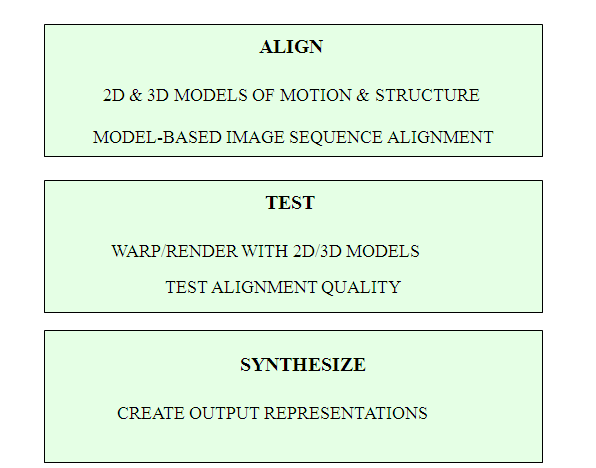# MATLAB VIDEO PROCESSING

Matlab Video Processing Projects require a stream processing in which video frames from a continuous stream are processes on or more at a time. Video processing is a signal processing which employs video filters, where the input and output signals are video files or video streams.

Uses of Commands and Math Functions in Matlab Video Processing:

Math functions are very much useful in developing Matlab video processing projects.

• Mathematical Calculations.
• Generating Plots.
• Perform numerical methods.• Completed Matlab Video Processing Projects 43%
• ON Going Matlab Video Processing Projects 17%

Elements of Matlab Programming:

• Flow control blocks.
• Scripts.
• Expressions.
• Functions.

Video Processing Performance:

• Aspect Ratio Control.
• Motion Compensation.
• Frame rate conversion.
• Detail Enhancement.

Code for Conversion in Matlab Video Processing.

% To get information about video file
file=aviinfo(‘test.avi’);
% To get the no of frames in the video file
frm_cnt=file.NumFrames
FileExtension=’.bmp’
%Progress bar starts
%Begin Loop process till End of Frames
for i=1:frm_cnt
% Read the Frame of the Video file
% Convert Frame to image file
frm_name=frame2im(frm(i));
%Create Filename to store
Filename=strcat(strcat(num2str(i)),FileExtension);
% Write image file to the current folder
imwrite(frm_name,Filename);
waitbar(i/frm_cnt,h)
end
%Close Progress bar
close(h)

Code for Motion Estimation in Matlab Video Processing.

Read and convert RGB image to grayscale
Create objects
htran = vision.GeometricTranslator(‘Offset’, [5 5], ‘OutputSize’, ‘Same as input image’);
hbm = vision.BlockMatcher(‘ReferenceFrameSource’, ‘Input port’, ‘BlockSize’, [35 35]);
hbm.OutputValue = ‘Horizontal and vertical components in complex form’;
halphablend = vision.AlphaBlender;
Offset the first image by [5 5] pixels to create second image
img2 = step(htran, img1);
Compute motion for the two images
motion = step(hbm, img1, img2);
Blend two images
img12 = step(halphablend, img2, img1);
Use quiver plot to show the direction of motion on the images[X Y] = meshgrid(1:35:size(img1, 2), 1:35:size(img1, 1));
imshow(img12);
hold on;
quiver(X(:), Y(:), real(motion(:)), imag(motion(:)), 0); hold off;